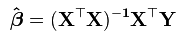# Matrix Algebra, Just Because

October 2, 2014 | 1 Comment

I was talking to a friend of mine today who had taken a test for a new job recently and he had a hard time with the math portion of it. We were in college about the same time and he did perfectly fine in math, but it had been a while. This got me to thinking that I should review things like matrix algebra from time to time, just because it has been a while since I had any need to multiply a matrix without a computer. Well, actually, I can’t imagine that I will ever have such a need but since I’m teaching multivariate statistics and the textbooks generally have a lot of matrix algebra, I thought I should brush up on it whether I ever need it or not.

I had the normal equations for regression drilled into my brain in graduate school and there was a time in my life when I actually had spare time when I found solving systems of linear equations something amusing to do. All of that was a very long time ago.

So …. as I sit here thinking what do my students need to know, I run into the Goldilocks problem yet again. Nothing seems just right. Teaching multiplying a scalar by a matrix seems a waste of time, no matter how brief. All you do is multiply every number in the matrix by that value. Okay, got it.

They should know what an Identity matrix is. This could actually have some useful implications in statistics. If your correlation matrix is close to an identity matrix, with 1 in the diagonals and 0s in the off-diagonal then it tells you that your variables are uncorrelated. If you analyzed a matrix of random data, this is exactly what you would expect to get.

If you multiply a matrix by the identity matrix, I, you are going to get the original matrix as a result, hence the name, identity matrix.

IA = A

This is analogous to the identity property of scalar (that is, regular numbers, not matrices) multiplication that 1X = X

The determinant of a matrix is, for a 2 x 2 matrix,  of this form

a b

c d

is equal to

To find the inverse of a matrix, the reciprocal of the determinant, that is 1 / (ad-bc), in the case of  our same 2 x 2 matrix is multiplied by the following matrix

d -b

c -a

Here is a really good Khan Academy video on finding the inverse of a matrix.

This is particularly important in statistics because you will occasionally get a message on your output that the “determinant is zero” and it would be helpful to you if you understood what that meant and why it was important.

One important point here is that you need the determinant to find the inverse of a matrix. For example, to find the vector of regression coefficients you would use this equationNotice here that you need to take the inverse of the product of the transpose of the X matrix and the X matrix. What if the determinant is zero? Well, you can’t divide by zero – SO THERE IS NO SOLUTION.

At this point, you want to start to chase down why the determinant is zero. Do you have redundant measures? Is there no variance in the sample?

All of this is very interesting to me personally, but aside from that, I keep asking myself whether the students really need an in-depth understanding of matrix algebra when it is all done by a computer. I really don’t know the answer to that, which is why I keep thinking about it.

Name (required)

Email (required)

Website

1 Comment so far

1. Ronald on October 2, 2014 12:00 pm

I recall that it’s almost never useful to find the inverse of a matrix – as long as you know that the matrix is invertible, doing the calculation with the original matrix is generally much easier than finding the inverse.

Usually, the importance of inverting matrices is over-emphasised.

But in fact, wikipedia lists your case as a (possible) exception to the rule: https://en.wikipedia.org/wiki/Invertible_matrix#Regression.2Fleast_squares